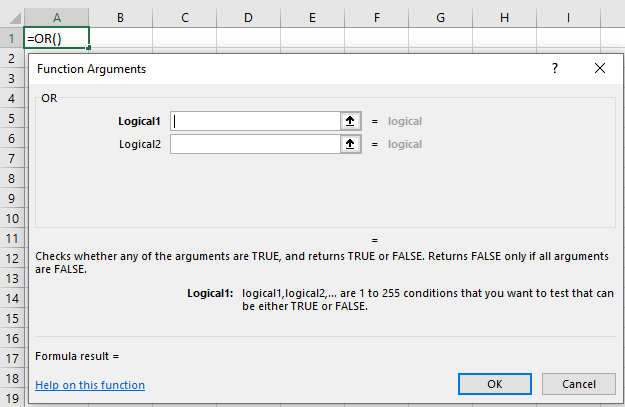# OR Function In ExcelArticle byExcelMojo Team## What Is OR Function In Excel?

The OR function in Excel returns TRUE if at least one of the conditions is true and returns FALSE if all are false. As a built-in Excel Logical function, the OR function makes other Excel functions involving logical tests more efficient.

For example, the table below has a list of fruits (column A) and the number of available boxes in the inventory (column B) and store (column C). So, let us check the availability status of the fruits in column D.

Using OR formula in Excel in column D can update the status for each fruit. The OR function compares two conditions in this example – whether the number of boxes in columns B or C is greater than 0. If one of the two conditions is TRUE, the status is TRUE; else, FALSE.

###### Key Takeaways
• The OR function in Excel helps the user compare multiple conditions simultaneously and returns TRUE or FALSE. It returns TRUE at least if one of the conditions is TRUE else it returns FALSE.
• The OR Excel formula is =OR(logicaltest1),[logicaltest2],…where,
• logical1: It is a mandatory argument and is the first logical value.
• logical2: It is an optional argument and is the second or the corresponding logical value.
• It assesses arguments and returns two logical values, TRUE or FALSE. The arguments can be references or arrays of logical value.
• You can use the OR() with other Excel functions involving logical evaluation, such as IF() and conditional formatting. The logical operator makes them highly efficient and more useful.

### OR() Excel Formula

The input arguments of the OR Excel formula are:

• logical1: It is a mandatory argument and refers to the first logical value to be tested.
• logical2: It is an optional argument; it denotes the second or the corresponding logical value.

### How To Use OR Excel Function?

Here are the steps to use the OR function in Excel:

1. Select the cell where you need to enter the OR() Excel formula.

2. Next, enter the ‘=’ sign and go to Formulas > Logical > OR.

3. The Function Arguments window appears. Then, enter the required arguments in the available box (Logical1 and Logical2).

4. Finally, click OK to view the OR() return value.

Alternatively, you can use the Formula dialog box to manually enter the required arguments for the OR function in Excel. Once you enter all the function arguments, press Enter key to get the result.

Let us understand the steps with an example of OR formula in Excel.

Consider the below table with a list of students and their overall aggregates.

We only need to confirm whether students have scored more than 90 marks. It is not required to know the students’ names or the actual scores obtained by them. We can use the OR function in Excel to update the needed results in cell D2.

Step 1: We will choose cell D2 to enter the OR().

Step 2: Next, we will go to Formulas > Logical > OR.

Step 3: The Function Arguments window pops up. Enter the logical_test value in the first argument box, Logical1, as B2:B11>90, and click OK.

Here we check if the values in the array or cell range B2:B11 are higher than 90. The OR function assesses the condition for each cell from B2:B11 and returns FALSE each time, as highlighted in the above image.

Please Note: When we enter the OR function in Excel arguments in the Function Arguments window, the OR Excel formula with the logical conditions gets updated in the target cell.

The output will be:

Please Note: We can manually enter the OR() Excel expression in cell D2 with the formula

=OR(B2:B11>90)

We will achieve the same output.

In the above example, as all the students have scored an aggregate less than 90, the condition evaluates to FALSE, and thus the OR() returns FALSE.

### Examples

Let us understand how the OR formula in Excel works with other Excel features and functions.

#### Example 1: Using With The Conditional Formatting Feature

Here is an example of OR function in Excel used with the conditional formatting Excel feature.

Consider the table depicted in the image below:

We need to determine the employees who did not swipe in/out on one or more days in Week 1. Then, we can use the OR function in Excel and apply conditional formatting to point out the employee names in the following steps:

Step 1: We will go to Home > Conditional Formatting > New Rule.

Step 2: The box for creating new Formatting Rules opens. Next, a formula will be used to choose which cells from the various alternatives should be formatted.

Step 3: Now, we will enter the OR() formula in the Format values where this formula is true to determine the employees who have not swiped on/off using the formula:

=OR(\$C3:\$H3=””)

And we will then click on Format to choose how we want to highlight the required names.

Step 4: The Format Cells tab appears. For this example, let us choose the font color Red from the Font tab.

Step 5: Now, we will click OK in the Format Cells window, and see the selected format in the New Formatting Rule box. Finally, we will choose OK to continue.

Step 6: Next, we will go to Home > Conditional Formatting > Manage Rules.

Step 7: The Conditional Formatting Rules Manager window opens up. With the settings shown in the image below, enter the cell range where we need the conditional formatting. In our example, it is \$A\$3:\$A\$12.

Step 8: Click OK to view the output.

Thus, we applied conditional formatting using the OR function in Excel. As a result, we can see that the employees who did not swipe in/out every day in Week 1 were highlighted in Red.

#### Example 2: Using With IF Function

Let us now understand how the OR formula with IF Excel formula.

Below is a table of a company’s branch-wise profit and sales data. We will try to update the bonus status for each branch office in column E.

The bonus status will be “APPROVED” if the specific branch has an operations team, the sales are over \$5000, or the profit is more than \$1000. So, let us see how OR functions in Excel with IF in the following steps.

Step 1: Choose cell E2 to enter the IF() containing the OR logical function.

Step 2: Enter the IF formula as shown below:

=IF(B2=”YES”,IF(OR(C2>5000,D2>1000),”APPROVED”,”NOT APPROVED”),”NA”)

Step 3: Press Enter to view the output.

Step 4: Select cell E2 and drag the fill handle downwards to copy the IF formula in all the cells from E3:E16.

Thus, we can see that OR function in Excel with IF formula has returned the bonus status as APPROVED to those branch offices that satisfied at least one of the logical tests in the OR().

Similarly, we can use multiple OR functions in an IF() and IF() with OR and AND operations.

### Important Things To Note

• You can test a maximum of 255 arguments (criteria) simultaneously in the OR function.
• The function ignores arguments with text values or blank cells.
• When using OR function in Excel, you may get the #VALUE! error. It occurs when any provided arguments do not interpret as a logical value.
• While the numbers in an OR() argument do not require a double quotation, a text string must be within a double quotation.

How to write OR function in Excel?

You can write OR function in Excel using the below steps:

1. Choose the cell where you want to enter the OR() formula.
2. Then, enter the ‘=’ sign, OR, ‘(‘ to write the function.
3. Next, enter the logical tests as the OR() arguments, separated by a comma.
4. Finally, enter ‘)’ and click on the Enter key to view the function return value.

How does OR function work in Excel?

The OR function compares multiple logical conditions and returns the function output as either TRUE or FALSE. If any provided condition is assessed as TRUE, the function returns TRUE. But if all arguments are FALSE, the function returns FALSE.

When to use OR function in Excel?

You can use OR function in Excel when you need to make a logical decision, TRUE or FALSE, based on checking multiple conditions simultaneously.

For example, consider the table of the list of awards won by various departments in the academic year 2021-2022. But, first, we need to find the number of departments that have won more than 3 awards.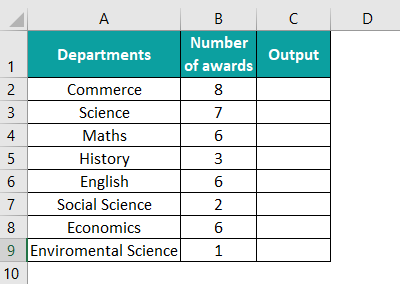We can use the OR function in Excel to display the results in cell C2.

Step 1: Choose cell C2 to enter the OR().

Step 2: Next, go to Formulas > Logical > OR.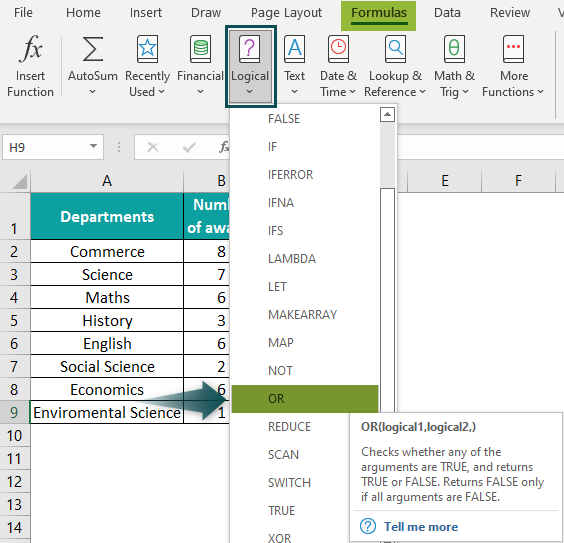Step 3: The Function Arguments window pops up. Enter the logical_test value in the first argument box, Logical1, as B2:B9>3, and click OK.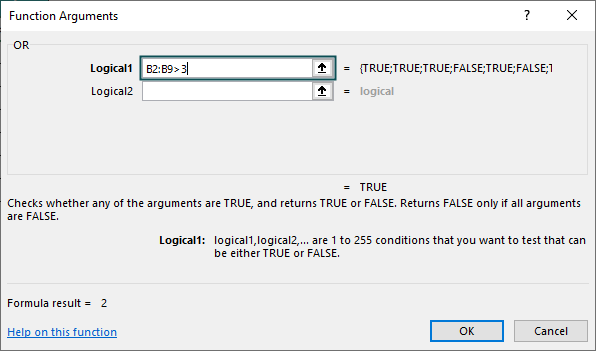The output will be returned in cell C2 as follows: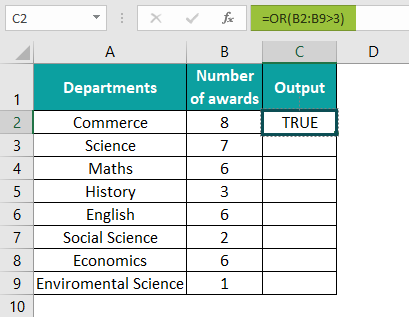Step 4: Select cell C2 and drag the fill handle downwards to get the results in cell range A3:A16, as shown below.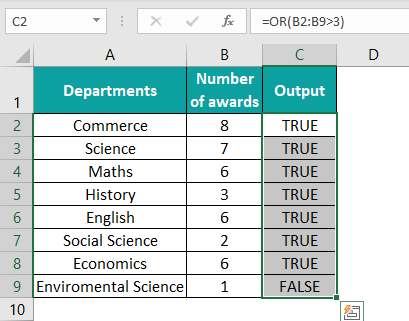Thus, the OR() function has returned the logical values as ‘TRUE’ and ‘FALSE,’ where the former indicates the departments having won more than 3 awards and the latter refers to those having won less than 3 awards.

You can also use it with other Excel functions and features involving logical tests, such as IF, AND, and conditional formatting.

Where is the OR function in Excel?

The OR function in Excel is in the Function Library group of the Formulas tab.

Step 1: Choose the required cell, and go to Formulas à Logical à OR to use the function.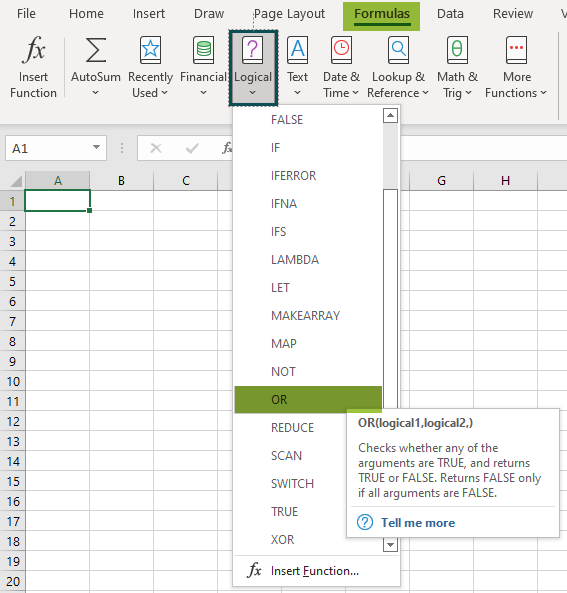Step 2: Once you click on OR(), the function will appear in the selected cell, and the Function Arguments window will open. You can enter the arguments here and click OK to perform your required calculations.